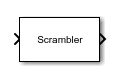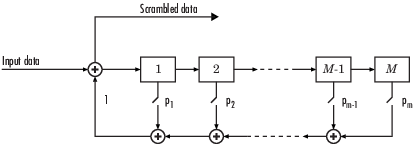# Scrambler

Scramble input signal

• Library:
• Communications Toolbox / Sequence Operations

•## Description

The Scrambler block scrambles a scalar or column vector input signal.

One purpose of scrambling is to reduce the length of consecutive 0s or 1s in a transmitted signal. Long sequences of 0s or 1s can cause transmission synchronization problems. This schematic shows the scrambler operation. All adders perform addition modulo N, where N is the value specified by the Calculation base parameter.At each time step, the input causes the contents of the registers to shift sequentially. Using the Scramble polynomial parameter, you specify the on or off state for each switch in the scrambler.

To achieve repeatable initial scrambler conditions, you can use one of these optional input ports:

This block can accept input sequences that vary in length during simulation. For more information about sequences that vary in length, see Variable-Size Signal Basics (Simulink).

## Ports

### Input

expand all

Input data signal, specified as an NS-by-1 vector. NS represents the number of samples in the input signal. The input values must be integers from `0` to Calculation base`1`.

Data Types: `double`

Reset scrambler, specified as a scalar. The scrambler is reset if a nonzero input is applied to the port.

#### Dependencies

To enable this port, set Initial states source to ```Dialog Parameter``` and select Reset on nonzero input via port.

Initial states of the scrambler registers when the simulation starts, specified as a nonnegative integer vector. The length of ISt must equal the order of the Scramble polynomial parameter. The vector element values must be integers from `0` to Calculation base`1`.

#### Dependencies

To enable this port, set Initial states source to ```Input port```.

### Output

expand all

Output scrambled data, returned as an NS-by-1 vector. NS equals the number of samples in the input signal.

Data Types: `double`

## Parameters

expand all

Calculation base used in the scrambler for modulo operations, specified as a nonnegative integer. The input and output of this block are integers from `0` to Calculation base`1`.

Polynomial that defines the connections in the scrambler, specified as a character vector, integer vector, or binary vector. The Scramble polynomial parameter defines if each switch in the scrambler is on or off. Specify the polynomial as:

• A character vector, such as ```'1 + z^-6 + z^-8'```. For more details on specifying polynomials in this way, see Character Representation of Polynomials.

• An integer vector, such as `[0 -6 -8]`, listing the descrambler coefficients in order of descending powers of z-1, where p(z-1) = 1 + p1z-1 + p2z-2 + ...

• A binary vector, such as ```[1 0 0 0 0 0 1 0 1]```, listing the powers of z that appear in the polynomial that has a coefficient of 1. In this case, the order of the scramble polynomial is one less than the binary vector length.

Example: `'1 + z^-6 + z^-8'`, ```[0 -6 -8]```, and `[1 0 0 0 0 0 1 0 1]` all represent this polynomial:

p(z-1) = 1 + z-6 + z-8

• `Dialog Parameter` – Specify scrambler initial states by using the Initial states parameter.

• `Input port` – Specify scrambler initial states by using the ISt port.

Initial states of scrambler registers when the simulation starts, specified as a nonnegative integer vector. The length of Initial states must equal the order of the Scramble polynomial parameter. The vector element values must be integers from `0` to Calculation base`1`.

#### Dependencies

This parameter is available when Initial states source is set to ```Dialog Parameter```.

Select this parameter to reset the Scrambler block via input port `Rst`.

#### Dependencies

This parameter is available when Initial states source is set to ```Dialog Parameter```.

## Block Characteristics

 Data Types `Boolean` | `double` | `integer` Multidimensional Signals `no` Variable-Size Signals `no`

## Version History

Introduced before R2006a